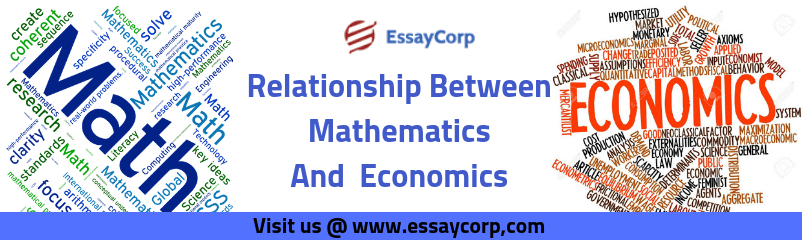# All About the Relationship Between Mathematics and EconomicsMathematics is considered to be an integral and fundamental part of economics. As it is a well-known fact that price and money are crucial aspects of the economy and as a result economics as well. It has been observed that most economists exercise mathematical paradigms to forecast and predict many things from the aspects like demand. Firstly we need to apprehend the meaning of mathematics and economics individually.

## Mathematics

We all are aware of mathematics but when it comes to defining this particular term it becomes quite tricky. Mathematics is simply the study of numbers, amounts, shapes, quantity, measurements, and their relationship with each other. Structures, conjectures, and Axioms are studied in the mathematics discipline. Though it is considered to be a difficult subject still it is popular among students.

## Economics

Economics is also a part of social science and it can be understood as the social science that analyzes, examine and illustrate the production, circulation, and exhaustion of resources. The study of economics depicts the process of demand-supply forces in the effective allocation of scarce resources. There are two main categories of this academic discipline i.e. and microeconomics.

#Numerous models are typically designed as functions. For instance, the demand for a product is a function of the price of that particular product or good, the cost of alternative goods or we can say substitute goods, and income. There tends to be a comprehensive usage of functions in economics. Calculus, linear algebra, etc. are the fundamental implements that are exercised by economists. Apart from these aspects, several statistical models and functions are used in certain economic analyses. “Econometrics” is a separate field in economics that deals with statistical functions.

Economic analysis generally exercises and uses the quantitative approach and method while assessing any particular information in an economy. Quantitative methods are considered to be the mathematical calculations that give pointers to analyze current and past events and economic periods. Numerous sorts of mathematics have been used by economists to make their studies, judgments, and theories more certain and reliable through apprehended calculations.

## Use of calculus in economics

Calculus is considered to be the most usual and common type of math that can be observed in economics. Calculus comprises the usage of many formulas to calculate the limits, operations, functions, and derivatives as well. The differential calculus has been exercised by many economists to assess certain information related to economics.

### The Overview

Economic analysis can prove to be beneficial in the numbers that are exercised in the equations of mathematics or the economic models related to it. The little deformations that are carried in these numbers can present some serious obstacles while apprehending the economic trends.